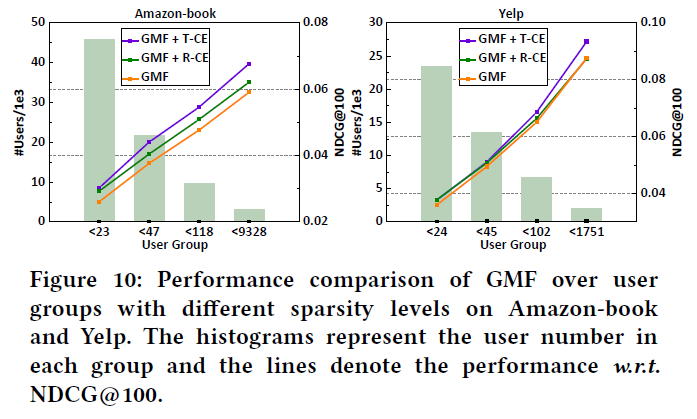5G时代下一个聚合的编程学习网

# Denoising Implicit Feedback for Recommendation

## INTRODUCTION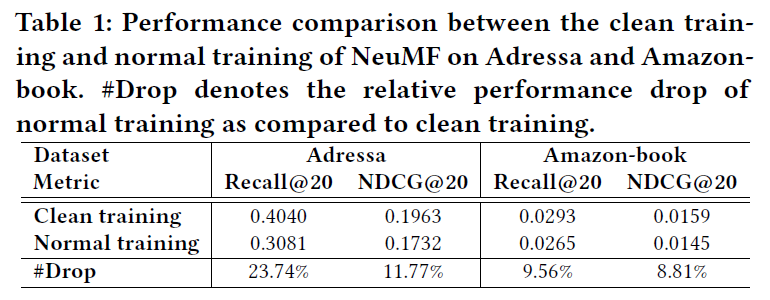• 1）负面经验识别,Figure 1(b)。可以通过附加的用户行为（例如，停留时间和凝视模式）和辅助的项目特征（例如，项目描述）
• 2）纳入各种反馈来消除,Figure 1(c)。将额外的反馈（例如，喜爱和跳过）合并到推荐器训练中，以剔除假阳性交互的影响。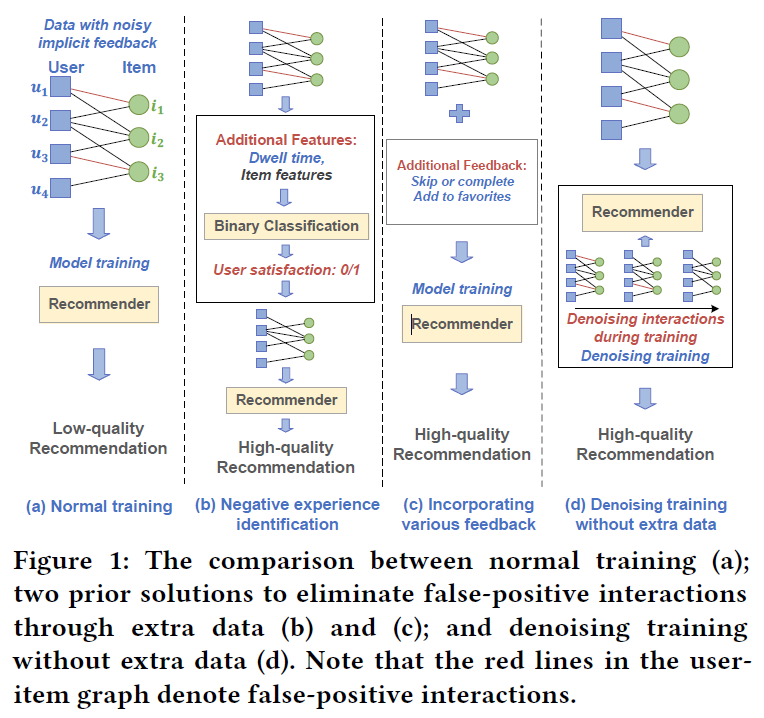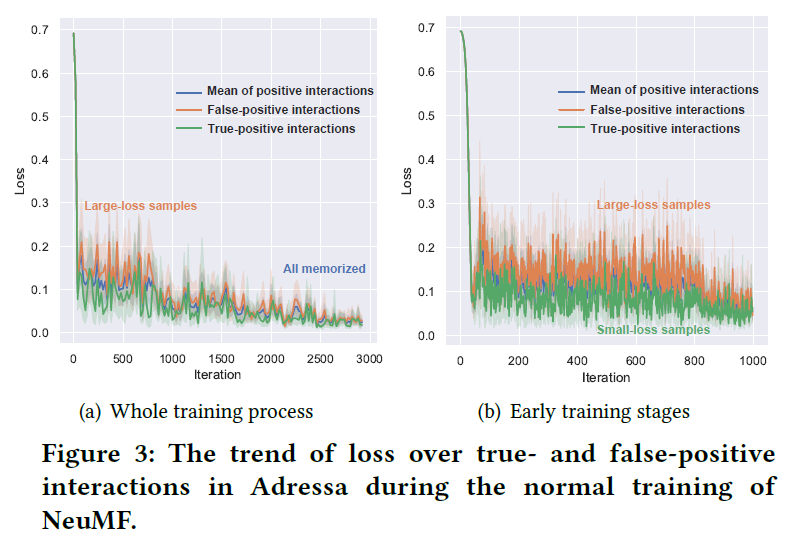• 假阳性互动更难适用于推荐器，在很大程度上会误导早期的培训目标。原因之一是假阳性交互代表用户不喜欢的项目，应该属于阴性样本；
• 推荐器由于其高表达性，最终能适合假阳性交互，这是过度拟合。

• 截断损失：通过动态阈值，来忽略大损失样本，这个阈值会动态更新；
• 重加权损失：动态分配较小权重给”harder”的交互，以削弱它们对模型优化的影响；

## STUDY ON FALSE-POSITIVE FEEDBACK

• Amazon-book：这是一个产品推荐数据集，其中包含的购买历史评分范围从1到5（5表示最好），直观的将评分低于3的用户-商品交互视为假阳性交互

Settings：

• “clean training”，它只在真阳性交互的数据集上训练NeuMF；
• “normal training”，在整体的数据集上训练NeuMF；

Results：

## METHOD

[mathcal{L}_{CE}(mathcal{D}^*)=-sum_{(u,i,y_{ui}^*)inmathcal{D}^*}y_{ui}^*log(hat{y}_{ui})+(1-y_{ui}^*)log(1-hat{y}_{ui})
]

(y_{ui}^*in {0,1})表示用户u是真实的喜欢项目i。带有(Theta^*)参数的推荐系统能够可靠地产生高质量推荐。实际上，由于缺乏大量可靠的反馈，推荐系统的训练杯形式化成

[ar{Theta}=minmathcal{L}_{CE}(ar{mathcal{D}})
]

[Theta^*=minmathcal{L}_{CE}(denoise(ar{mathcal{D}}))
]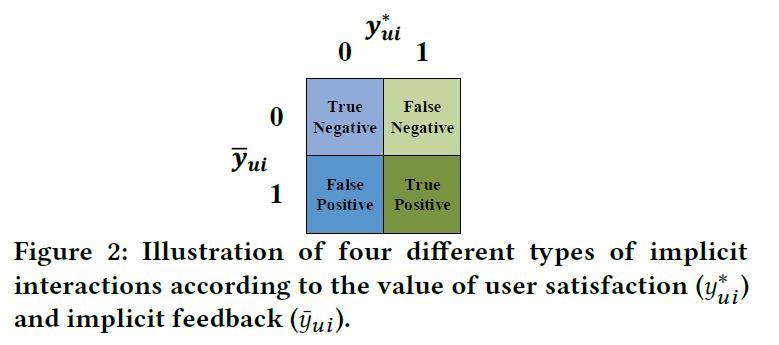3.2 Observations• 真阳性和假阳性交互的损失都会收敛到具有接近值的稳定状态，这说明NeuMF适合模拟这两个状态。同时也反映了深度学习的模型的模拟能力很强大，可以同时模拟正确和错误的标签样本。因此，如果训练数据有噪声，会导致较差的泛化能力；
• 在训练过程的早期，真阳性和假阳性交互的损失值有不同程度的下降。可以看出假阳性交互的损失明显大于真阳性交互的损失。真阳性交互的损失较小，意味着相对比较容易模拟。而损失较大的假阳性会更难模拟，原因是假阳性表示了用户不喜欢的项目。

• Truncated Loss（截断损失）：利用动态阈值函数将大损失交互的损失值截断为0；
• Reweighted Loss（重新加权损失）：在训练过程中自适应地给harder样本分配较小的权重；

3.3.1 Truncated Cross-Entropy Loss

[mathcal{L}_{T-CE}(u,i)=egin{cases}
0,&mathcal{L}_{CE}(u,i)> au land ar{y}_{ui}=1 \
mathcal{L}_{CE}(u,i), & otherwise,
end{cases}
]

• (epsilon(cdot))应该有一个上限来限制丢弃样本的比例，以防止数据丢失；
• (epsilon(0)=0)，允许所有样本在开始时输入模型；
• (epsilon(cdot))应该从0平滑地增加到上限，以便魔心可以逐渐学习和区分真假阳性交互；

[epsilon(T)=min(alpha T,epsilon_{max})
]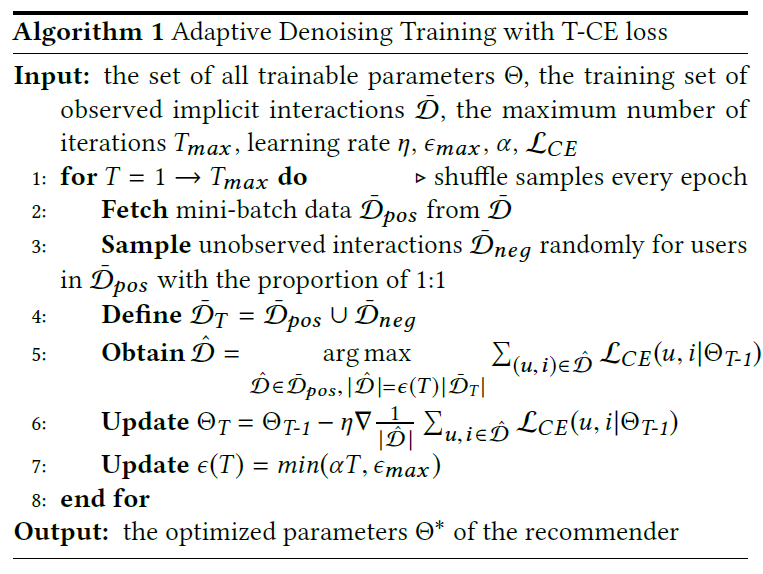3.3.2 Reweighted Cross-Entropy Loss

[mathcal{L}_{R-CE}(u,i)=omega (u,i)mathcal{L}_{CE}(u,i)
]

• 该函数在训练期间动态调整样本的权重
• 该函数将harder样本（大损失交互的样本）的影响降低到比简单样本弱的程度；
• 权重减轻的程度可以简单的调整，以便可以适应不同的模型和数据集

[f(hat{y}_{ui})=hat{y}_{ui}^{eta}
]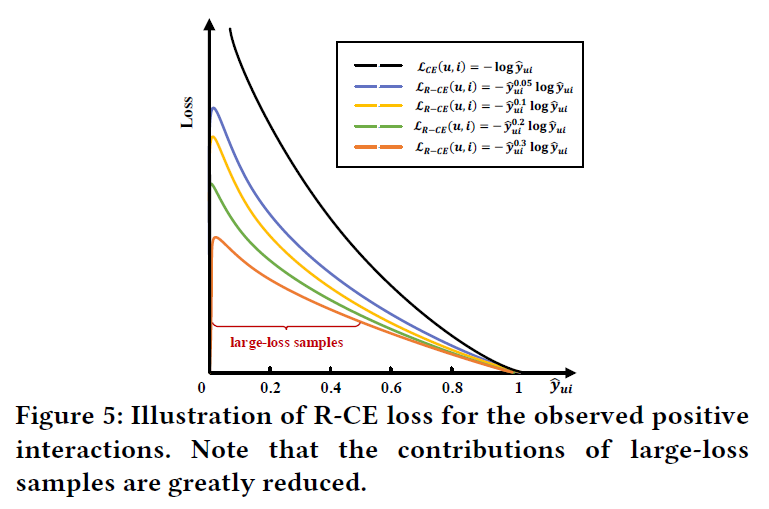• (f(hat{y}_{ui})=hat{y}_{ui}^{eta})对于损失值相比(hat{y}_{ui})更加敏感。因此，它可以在训练过程中生成动态权重；
• 具有极大损失的交互（例如图6的“outlier”——异常值）将被分配非常小的权重，因为(hat{y}_{ui})接近于0。因此，大损失样本的影响大大降低。从下图可以看出，较难的样本总是具有较小的权重，不过困难样本和简单样本的权重都降低。这是因为当(hat{y}_{ui}in [0,1]),(eta in [0,+infty])函数(f(hat{y}_{ui})=hat{y}_{ui}^{eta})是单调递增的。harder样本的预测评分比easy样本的评分低。因此，可以避免具有大损失值的假阳性样本在优化过程中占主导作用。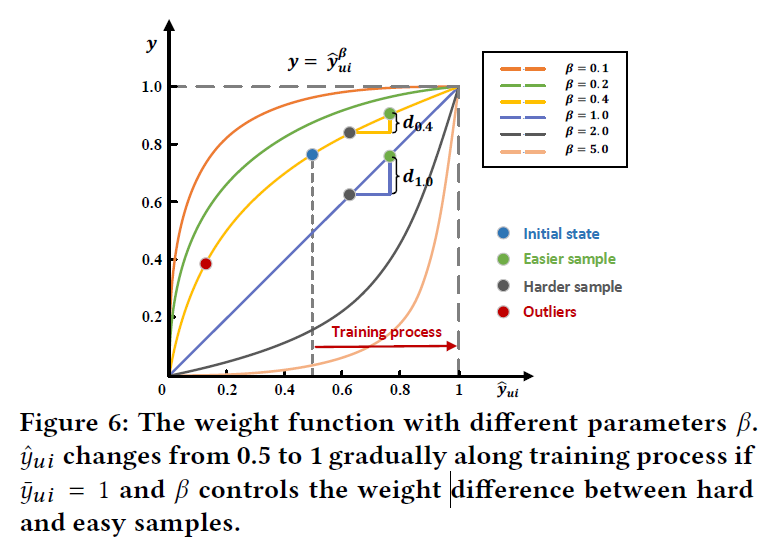• 超参数(eta)在训练过程中，动态地控制困难交互和简单交互之间的gap，上图绘制了各种(eta)设置下的权重函数(f(hat{y}_{ui})=hat{y}_{ui}^{eta})。在训练过程中，预测分数(hat{y}_{ui})在初始迭代中波动在0.5左右，然后逐渐移动到1。在图6中，绘制了四种情况，initial states，easier sample，harder sample，outlier。从图中可以看出：
• 1）(hat{y}_{ui}^eta)是递增的
• 2）(eta)的增大，对于同一对简单和困难的样本，它们之间的权重差距会变得更大，如图中的(d_{0.4}<d_{1.0})。此外，如果将(eta)设置为0，R-CE损失退化成标准的CE损失。

[omega(u,i)=egin{cases}
hat{y}_{ui}^eta, & ar{y}_{ui}=1\
(1-hat{y}_{ui})^eta,& otherwise
end{cases}
]

[frac{partial mathcal{L}_{R-CE}}{partial Theta}=frac{partial mathcal{L}_{R-CE}}{partial hat{y}_{ui}}frac{partialhat{y}_{ui}}{partialTheta}=-hat{y}_{ui}^etafrac{1}{hat{y}_{ui}}frac{partialhat{y}_{ui}}{partialTheta}=hat{y}_{ui}^{eta}(frac{partial mathcal{L}_{CE}}{partial hat{y}_{ui}}frac{partial hat{y}_{ui}}{partial Theta})
]

## EXPERIMENT

Dataset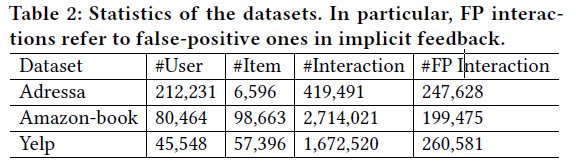• Amazon-book：此数据集包括用户对其购买的书的评分；
• Yelp：用户对餐饮业的评分，其中评分低于3分被视为假阳性反馈；

Evaluation Protocols

Testing Recommenders

• GMF：矩阵分解的广义版本，将内积替换为逐元素乘积和线性神经层作为交互函数；
• NeuMF：NeuMF是具有代表性的CF神经模型，它通过结合GMF和多层感知器（MLP）对用户和项目之间的关系进行建模；
• CDAE：用随机噪声破坏观察到的交互作用，然后采用MLP模型重建原始交互，部分提高了抗噪声能力；

Parameter Settings

ADT策略有三个超参数：在T-CE loss中的(alpha,epsilon_{max})，在R-CE loss中的(eta)(epsilon_{max})在{0.05, 0.1, …, 0.5}中搜索，(eta)在{0.05, 0.1, …, 0.25, 0.5, 1.0}中调整，控制(alpha)的范围，通过迭代次数(epsilon_N)调整到最大drop rate：(epsilon_{max})(epsilon_N)在{1k, 5k, 10k, 20k, 30k}中选取；

5.1 Overall Performance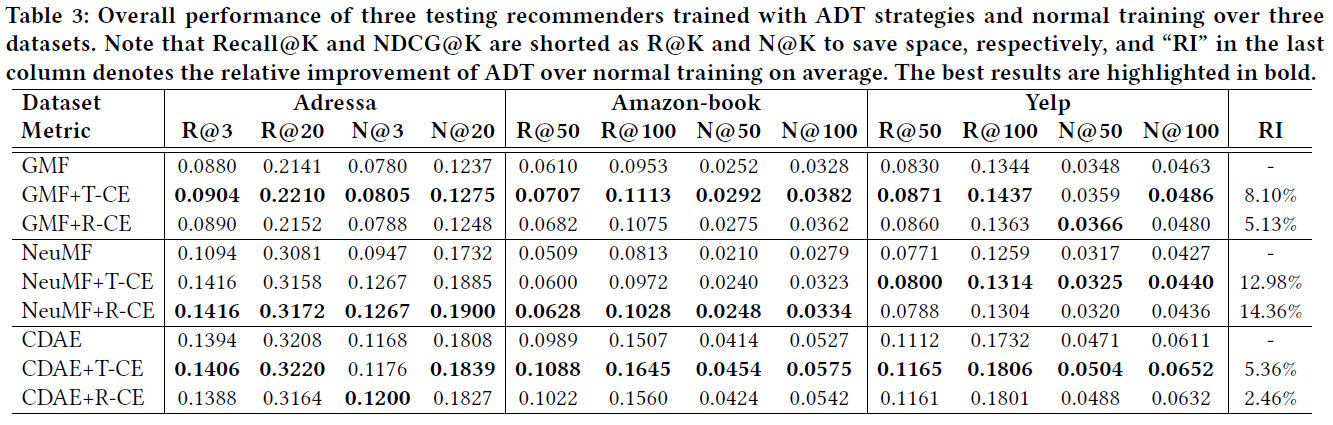• 通过比较T-CE和R-CE，可以看出T-CE的表现更好。这是因为R-CE仍然受到假阳性交互的影响，即使它们具有较小的权重。此外，T-CE中的动态阈值函数可以通过两个超参数进行更精确的调整；

5.2 Discussion

5.2.1 Memorization of False-positive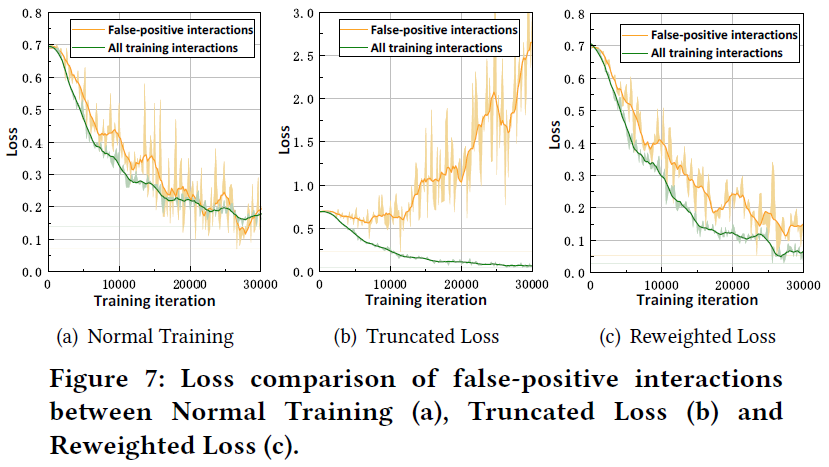• 假阳性交互的损失值最终变得与其他样本相似，表明GMF最终能很好地记住假阳性样本；
• 当GMF用T-CE loss训练时，假阳性交互的损失值变得非常大，而所有训练样本的损失值都稳定且很小。这是因为推荐器总是选择损失小的样本来优化参数，越来越多的噪声交互在训练过程中没有拟合；
• 当使用R-CE损失训练GMF时，假阳性交互的损失也随着训练过程而减少。

5.2.2 Study of Truncated Loss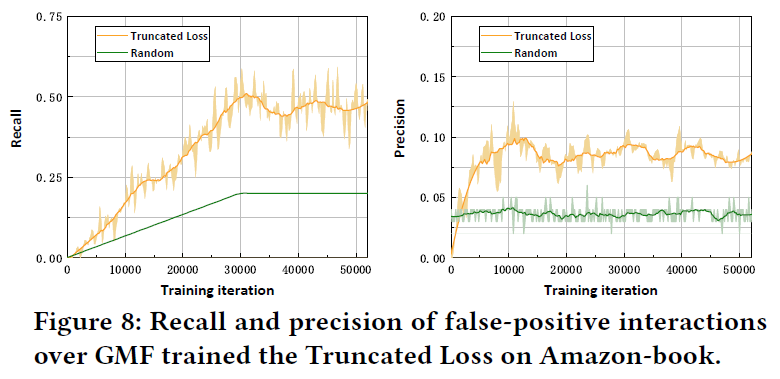• 在drop rate稳定后，截断损失丢弃几乎一半的假阳性交互；大大地降低了噪声交互的影响；
• 截断损失的精度大约是随机丢弃精度的两倍。它表明截断损失有效地利用了假阳性交互的信号，并削弱了它们对模型训练的影响；

5.2.3 Hyper-parameter Sensitivity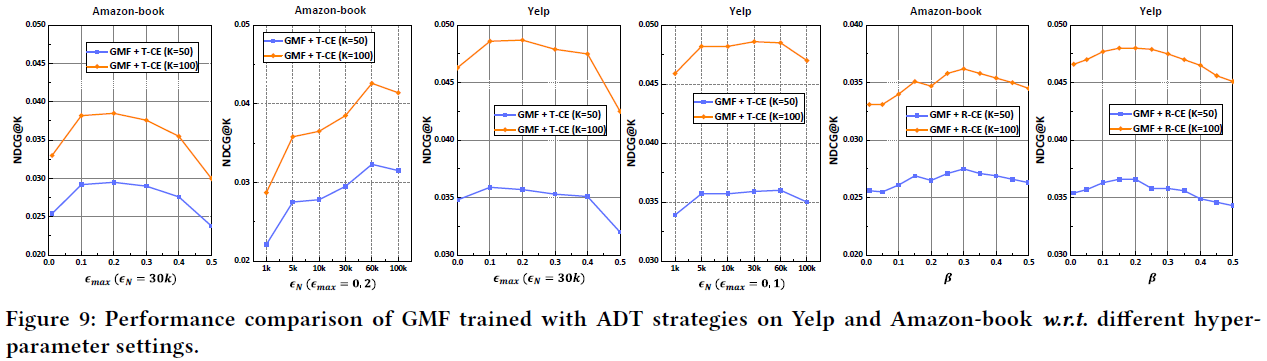• (epsilon_{max}in [0.1, 0.3])时，使用T-CE损失训练的推荐模型实现了最大的性能提升。如果(epsilon_{max})超过0.4，性能会显著下降。因为丢弃了很大比例的样本。因此，截断损失的上限(epsilon_{max})应该受到限制；
• 推荐模型与(epsilon_{N})的关系更密切，尤其是在Amazon-book上，当(epsilon_{N})大于30k时了，性能仍然提高。但限制是大搜索空间限制了超参数调整；
• 重加权损失中的(eta)的调整在不同的数据集上是一致的，当(eta)的范围在0.15到0.3时，效果最好。

5.2.4 Performance Comparison w.r.t. Interaction Sparsity